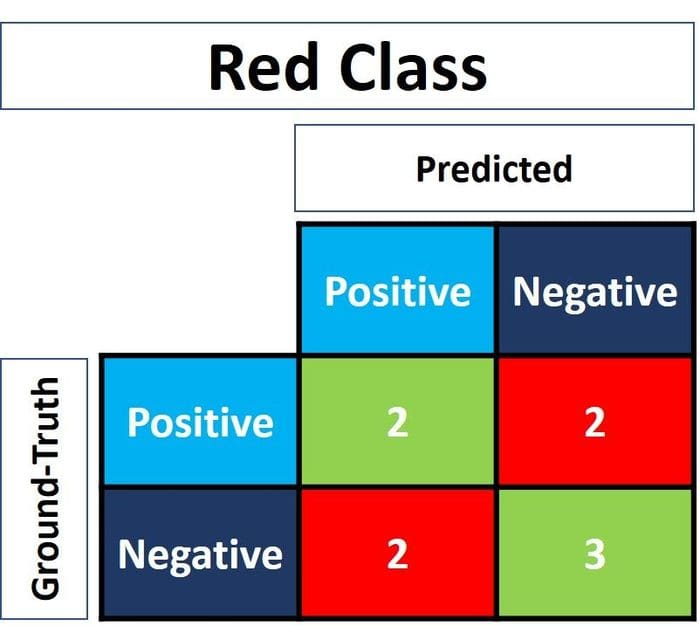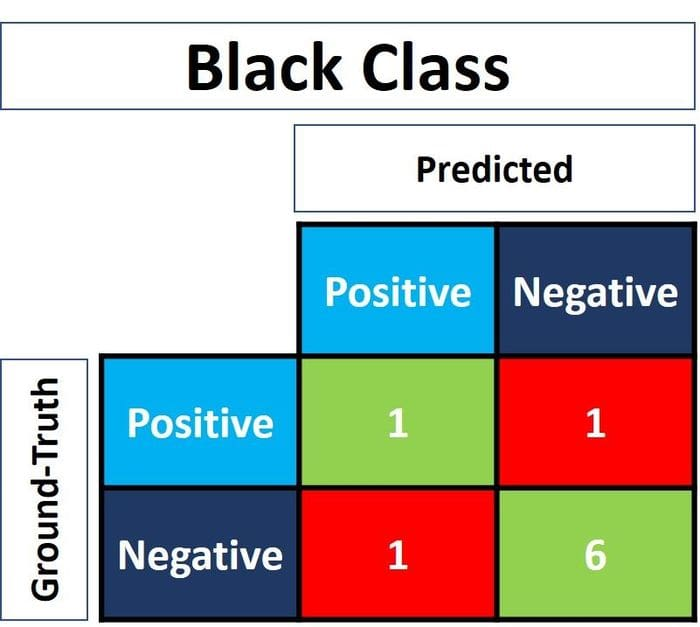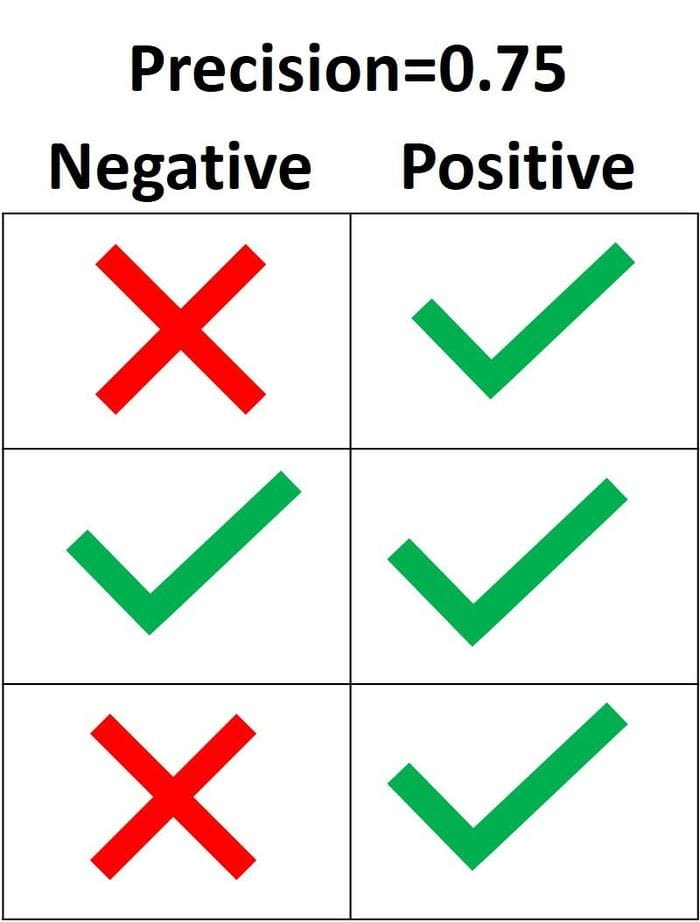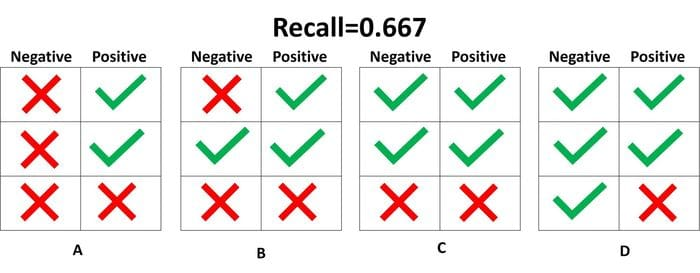Submit a blog to KDnuggets -- Top Blogs Win A Reward

Topics: AI | Data Science | Data Visualization | Deep Learning | Machine Learning | NLP | Python | R | Statistics

KDnuggets Home » News » 2021 » Feb » Tutorials, Overviews » Evaluating Deep Learning Models: The Confusion Matrix, Accuracy, Precision, and Recall ( 21:n08 )

#Evaluating Deep Learning Models: The Confusion Matrix, Accuracy, Precision, and Recall

This tutorial discusses the confusion matrix, and how the precision, recall and accuracy are calculated, and how they relate to evaluating deep learning models.

In computer vision, object detection is the problem of locating one or more objects in an image. Besides the traditional object detection techniques, advanced deep learning models like R-CNN and YOLO can achieve impressive detection over different types of objects. These models accept an image as the input and return the coordinates of the bounding box around each detected object.

This tutorial discusses the confusion matrix, and how the precision, recall and accuracy are calculated. In another tutorial, the mAP will be discussed.

Specifically, we'll cover:

• Confusion Matrix for Binary Classification
• Confusion Matrix for Multi-Class Classification
• Calculating the Confusion Matrix with Scikit-learn
• Accuracy, Precision, and Recall
• Precision or Recall?
• Conclusion

### Confusion Matrix for Binary Classification

In binary classification each input sample is assigned to one of two classes. Generally these two classes are assigned labels like 1 and 0, or positive and negative. More specifically, the two class labels might be something like malignant or benign (e.g. if the problem is about cancer classification), or success or failure (e.g. if it is about classifying student test scores).

Assume there is a binary classification problem with the classes positive and negative. Here is an example of the labels for seven samples used to train the model. These are called the ground-truth labels of the sample.

``positive, negative, negative, positive, positive, positive, negative``

Note that the class labels are used to help us humans differentiate between the different classes. The thing that is of high importance to the model is a numeric score. When feeding a single sample to the model, the model does not necessarily return a class label, but rather a score. For instance, when these seven samples are fed to the model, their class scores could be:

``0.6, 0.2, 0.55, 0.9, 0.4, 0.8, 0.5``

Based on the scores, each sample is given a class label. How do we convert these scores into labels? We do this by using a threshold. This threshold is a hyperparameter of the model and can be defined by the user. For example, the threshold could be 0.5–then any sample above or equal to 0.5 is given the positive label. Otherwise, it is negative. Here are the predicted labels for the samples:

``positive (0.6), negative (0.2), positive (0.55), positive (0.9), negative (0.4), positive (0.8), positive (0.5)``

For comparison, here are both the ground-truth and predicted labels. At first glance we can see 4 correct and 3 incorrect predictions. Note that changing the threshold might give different results. For example, setting the threshold to 0.6 leaves only two incorrect predictions.

``````Ground-Truth: positive, negative, negative, positive, positive, positive, negative
Predicted   : positive, negative, positive, positive, negative, positive, positive``````

To extract more information about model performance the confusion matrix is used. The confusion matrix helps us visualize whether the model is "confused" in discriminating between the two classes. As seen in the next figure, it is a 2×2 matrix. The labels of the two rows and columns are Positive and Negative to reflect the two class labels. In this example the row labels represent the ground-truth labels, while the column labels represent the predicted labels. This could be changed.The 4 elements of the matrix (the items in red and green) represent the 4 metrics that count the number of correct and incorrect predictions the model made. Each element is given a label that consists of two words:

1. True or False
2. Positive or Negative

It is True when the prediction is correct (i.e. there is a match between the predicted and ground-truth labels), and False when there is a mismatch between the predicted and ground-truth labels. Positive or Negative refers to the predicted label.

In summary, whenever the prediction is wrong, the first word is False. Otherwise, it is True. The goal is to maximize the metrics with the word True (True Positive and True Negative), and minimize the other two metrics (False Positive and False Negative). The four metrics in the confusion matrix are thus:

1. Top-Left (True Positive): How many times did the model correctly classify a Positive sample as Positive?
2. Top-Right (False Negative): How many times did the model incorrectly classify a Positive sample as Negative?
3. Bottom-Left (False Positive): How many times did the model incorrectly classify a Negative sample as Positive?
4. Bottom-Right (True Negative): How many times did the model correctly classify a Negative sample as Negative?

We can calculate these four metrics for the seven predictions we saw previously. The resulting confusion matrix is given in the next figure.This is how the confusion matrix is calculated for a binary classification problem. Now let's see how it would be calculated for a multi-class problem.

### Confusion Matrix for Multi-Class Classification

What if we have more than two classes? How do we calculate these four metrics in the confusion matrix for a multi-class classification problem? Simple!

Assume there are 9 samples, where each sample belongs to one of three classes: WhiteBlack, or Red. Here is the ground-truth data for the 9 samples.

``Red, Black, Red, White, White, Red, Black, Red, White``

When the samples are fed into a model, here are the predicted labels.

``Red, White, Black, White, Red, Red, Black, White, Red``

For easier comparison, here they are side-by-side.

``````Ground-Truth: Red, Black, Red,   White, White, Red, Black, Red,   White
Predicted:    Red, White, Black, White, Red,   Red, Black, White, Red``````

Before calculating the confusion matrix a target class must be specified. Let's set the Red class as the target. This class is marked as Positive, and all other classes are marked as Negative.

``````Positive, Negative, Positive, Negative, Negative, Positive, Negative, Positive, Negative
Positive, Negative, Negative, Negative, Positive, Positive, Negative, Negative, Positive``````

Now there are only two classes again (Positive and Negative). Thus, the confusion matrix can be calculated as in the previous section. Note that this matrix is just for the Red class.For the White class, replace each of its occurrences as Positive and all other class labels as Negative. After replacement, here are the ground-truth and predicted labels. The next figure shows the confusion matrix for the White class.

``````Negative, Negative, Negative, Positive, Positive, Negative, Negative, Negative, Positive
Negative, Positive, Negative, Positive, Negative, Negative, Negative, Positive, Negative``````Similarly, here is the confusion matrix for the Black class.### Calculating the Confusion Matrix with Scikit-Learn

The popular Scikit-learn library in Python has a module called `metrics` that can be used to calculate the metrics in the confusion matrix.

For binary-class problems the `confusion_matrix()` function is used. Among its accepted parameters, we use these two:

1. `y_true`: The ground-truth labels.
2. `y_pred`: The predicted labels.

The following code calculates the confusion matrix for the binary classification example we discussed previously.

``````import sklearn.metrics

y_true = ["positive", "negative", "negative", "positive", "positive", "positive", "negative"]
y_pred = ["positive", "negative", "positive", "positive", "negative", "positive", "positive"]

r = sklearn.metrics.confusion_matrix(y_true, y_pred)
print(r)``````

``````array([[1, 2],
[1, 3]], dtype=int64)``````

Note that the order of the metrics differ from that discussed previously. For example, the True Positive metric is at the bottom-right corner while True Negative is at the top-left corner. To fix that, we can flip the matrix.

``````import numpy

r = numpy.flip(r)
print(r)``````

``````array([[3, 1],
[2, 1]], dtype=int64)``````

To calculate the confusion matrix for a multi-class classification problem the `multilabel_confusion_matrix()` function is used, as shown below. In addition to the `y_true` and `y_pred` parameters, a third parameter named `labels` accepts a list of the class labels.

``````import sklearn.metrics
import numpy

y_true = ["Red", "Black", "Red",   "White", "White", "Red", "Black", "Red",   "White"]
y_pred = ["Red", "White", "Black", "White", "Red",   "Red", "Black", "White", "Red"]

r = sklearn.metrics.multilabel_confusion_matrix(y_true, y_pred, labels=["White", "Black", "Red"])
print(r)``````

``````array([
[[4 2]
[2 1]]

[[6 1]
[1 1]]

[[3 2]
[2 2]]], dtype=int64)``````

The function calculates the confusion matrix for each class and returns all the matrices. The order of the matrices match the order of the labels in the `labels` parameter.  To adjust the order of the metrics in the matrices, we'll use the `numpy.flip()` function, as before.

``````print(numpy.flip(r)) # White class confusion matrix
print(numpy.flip(r)) # Black class confusion matrix
print(numpy.flip(r)) # Red class confusion matrix``````

``````# White class confusion matrix
[[1 2]
[2 4]]

# Black class confusion matrix
[[1 1]
[1 6]]

# Red class confusion matrix
[[2 2]
[2 3]]``````

In the rest of this tutorial we'll focus on just two classes. The next section discusses three key metrics that are calculated based on the confusion matrix.

### Accuracy, Precision, and Recall

The confusion matrix offers four different and individual metrics, as we've already seen. Based on these four metrics, other metrics can be calculated which offer more information about how the model behaves:

1. Accuracy
2. Precision
3. Recall

The next subsections discuss each of these three metrics.

### Accuracy

Accuracy is a metric that generally describes how the model performs across all classes. It is useful when all classes are of equal importance. It is calculated as the ratio between the number of correct predictions to the total number of predictions.Here is how to calculate the accuracy using Scikit-learn, based on the confusion matrix previously calculated. The variable `acc` holds the result of dividing the sum of True Positives and True Negatives over the sum of all values in the matrix. The result is `0.5714`, which means the model is `57.14%` accurate in making a correct prediction.

``````import numpy
import sklearn.metrics

y_true = ["positive", "negative", "negative", "positive", "positive", "positive", "negative"]
y_pred = ["positive", "negative", "positive", "positive", "negative", "positive", "positive"]

r = sklearn.metrics.confusion_matrix(y_true, y_pred)

r = numpy.flip(r)

acc = (r + r[-1][-1]) / numpy.sum(r)
print(acc)``````

``0.571``

The `sklearn.metrics` module has a function called `accuracy_score()` that can also calculate the accuracy. It accepts the ground-truth and predicted labels as arguments.

``acc = sklearn.metrics.accuracy_score(y_true, y_pred)``

Note that the accuracy may be deceptive. One case is when the data is imbalanced. Assume there are a total of 600 samples, where 550 belong to the Positive class and just 50 to the Negative class. Since most of the samples belong to one class, the accuracy for that class will be higher than for the other.

If the model made a total of 530/550 correct predictions for the Positive class, compared to just 5/50 for the Negative class, then the total accuracy is `(530 + 5) / 600 = 0.8917`. This means the model is 89.17% accurate. With that in mind, you might think that for any sample (regardless of its class) the model is likely to make a correct prediction 89.17% of the time. This is not valid, especially when you consider the Negative class for which the model performed badly.

### Precision

The precision is calculated as the ratio between the number of Positive samples correctly classified to the total number of samples classified as Positive (either correctly or incorrectly). The precision measures the model's accuracy in classifying a sample as positive.When the model makes many incorrect Positive classifications, or few correct Positive classifications, this increases the denominator and makes the precision small. On the other hand, the precision is high when:

1. The model makes many correct Positive classifications (maximize True Positive).
2. The model makes fewer incorrect Positive classifications (minimize False Positive).

Imagine a man who is trusted by others; when he predicts something, others believe him. The precision is like this man. When the precision is high, you can trust the model when it predicts a sample as Positive. Thus, the precision helps to know how the model is accurate when it says that a sample is Positive.

Based on the previous discussion, here is a definition of precision:

The precision reflects how reliable the model is in classifying samples as Positive.

In the next figure, the green mark means a sample is classified as Positive and a red mark means the sample is Negative. The model correctly classified two Positive samples, but incorrectly classified one Negative sample as Positive. Thus, the True Positive rate is 2 and the False Positive rate is 1, and the precision is `2/(2+1)=0.667`. In other words, the trustiness percentage of the model when it says that a sample is Positive is 66.7%.The goal of the precision is to classify all the Positive samples as Positive, and not misclassify a negative sample as PositiveAccording to the next figure, if all the three Positive samples are correctly classified but one Negative sample is incorrectly classified, the precision is `3/(3+1)=0.75`. Thus, the model is 75% accurate when it says that a sample is positive.The only way to get 100% precision is to classify all the Positive samples as Positive, in addition to not misclassifying a Negative sample as Positive.In Scikit-learn, the `sklearn.metrics` module has a function named `precision_score()` which accepts the ground-truth and predicted labels and returns the precision. The `pos_label` parameter accepts the label of the Positive class. It defaults to `1`.

``````import sklearn.metrics

y_true = ["positive", "positive", "positive", "negative", "negative", "negative"]
y_pred = ["positive", "positive", "negative", "positive", "negative", "negative"]

precision = sklearn.metrics.precision_score(y_true, y_pred, pos_label="positive")
print(precision)``````

``0.6666666666666666``

### Recall

The recall is calculated as the ratio between the number of Positive samples correctly classified as Positive to the total number of Positive samples. The recall measures the model's ability to detect Positive samples. The higher the recall, the more positive samples detected.The recall cares only about how the positive samples are classified. This is independent of how the negative samples are classified, e.g. for the precision. When the model classifies all the positive samples as Positive, then the recall will be 100% even if all the negative samples were incorrectly classified as Positive. Let's look at some examples.

In the next figure, there are 4 different cases (A to D) and all have the same recall which is `0.667`. Each case differs only in how the negative samples are classified. For example, case A has all the negative samples correctly classified as Negative, but case D misclassifies all the negative samples as Positive. Independently of how the negative samples are classified, the recall only cares about the positive samples.Out of the 4 cases shown above, only 2 positive samples are classified correctly as positive. Thus, the True Positive rate is 2. The False Negative rate is 1 because just a single positive sample is classified as negative. As a result, the recall is `2/(2+1)=2/3=0.667`.

Because it does not matter whether the negative samples are classified as positive or negative, it is better to neglect the negative samples altogether as shown in the next figure. You only need to consider the positive samples when calculating the recall.What does it mean when the recall is high or low? When the recall is high, it means the model can classify all the positive samples correctly as Positive. Thus, the model can be trusted in its ability to detect positive samples.

In the next figure the recall is 1.0 because all the positive samples were correctly classified as Positive. The True Positive rate is 3, and the False Negative rate is 0. Thus, the recall is equal to `3/(3+0)=1`. This means the model detected all the positive samples. Because the recall neglects how the negative samples are classified, there could still be many negative samples classified as positive (i.e. a high False Positive rate). The recall doesn't take this into account.On the other hand, the recall is 0.0 when it fails to detect any positive sample. In the next figure all the positive samples are incorrectly classified as Negative. This means the model detected 0% of the positive samples. The True Positive rate is 0, and the False Negative rate is 3. Thus, the recall is equal to `0/(0+3)=0`.When the recall has a value between 0.0 and 1.0, this value reflects the percentage of positive samples the model correctly classified as Positive. For example, if there are 10 positive samples and the recall is 0.6, this means the model correctly classified 60% of the positive samples (i.e. `0.6*10=6` positive samples are correctly classified).

Similar to the `precision_score()` function, the `recall_score()` function in the `sklearn.metrics` module calculates the recall. The next block of code shows an example.

``````import sklearn.metrics

y_true = ["positive", "positive", "positive", "negative", "negative", "negative"]
y_pred = ["positive", "positive", "negative", "positive", "negative", "negative"]

recall = sklearn.metrics.recall_score(y_true, y_pred, pos_label="positive")
print(recall)``````

``0.6666666666666666``

After defining both the precision and the recall, let's have a quick recap:

• The precision measures the model trustiness in classifying positive samples, and the recall measures how many positive samples were correctly classified by the model.
• The precision takes into account how both the positive and negative samples were classified, but the recall only considers the positive samples in its calculations. In other words, the precision is dependent on both the negative and positive samples, but the recall is dependent only on the positive samples (and independent of the negative samples).
• The precision considers when a sample is classified as Positive, but it does not care about correctly classifying all positive samples. The recall cares about correctly classifying all positive samples, but it does not care if a negative sample is classified as positive.
• When a model has high recall but low precision, then the model classifies most of the positive samples correctly but it has many false positives (i.e. classifies many Negative samples as Positive). When a model has high precision but low recall, then the model is accurate when it classifies a sample as Positive but it can only classify a few positive samples.

Here are some questions to test your understanding:

1. If the recall is 1.0 and the dataset has 5 positive samples, how many positive samples were correctly classified by the model? (`5`)
2. Given that the recall is 0.3 when the dataset has 30 positive samples, how many positive samples were correctly classified by the model? (`0.3*30=9` samples)
3. If the recall is 0.0 and the dataset has 14 positive samples, how many positive samples were correctly classified by the model? (`0`)

### Precision or Recall?

The decision of whether to use precision or recall depends on the type of problem being solved. If the goal is to detect all the positive samples (without caring whether negative samples would be misclassified as positive), then use recall. Use precision if the problem is sensitive to classifying a sample as Positive in general, i.e. including Negative samples that were falsely classified as Positive.

Imagine that you are given an image and asked to detect all the cars within it. Which metric do you use? Because the goal is to detect all the cars, use recall. This may misclassify some objects as cars, but it eventually will work towards detecting all the target objects.

Now say you're given a mammography image, and you are asked to detect whether there is cancer or not. Which metric do you use? Because it is sensitive to incorrectly identifying an image as cancerous, we must be sure when classifying an image as Positive (i.e. has cancer). Thus, precision is the preferred metric.

### Conclusion

This tutorial discussed the confusion matrix and how to calculate its 4 metrics (true/false positive/negative) in both binary and multiclass classification problems. Using the `metrics` module in Scikit-learn, we saw how to calculate the confusion matrix in Python.

Based on these 4 metrics we dove into a discussion of accuracy, precision, and recall. Each metric is defined based on several examples. The `sklearn.metrics` module is used to calculate each of them.

Based on the concepts presented here, in the next tutorial we'll see how to use the precision-recall curve, average precision, and mean average precision (mAP).

Bio: Ahmed Gad received his B.Sc. degree with excellent with honors in information technology from the Faculty of Computers and Information (FCI), Menoufia University, Egypt, in July 2015. For being ranked first in his faculty, he was recommended to work as a teaching assistant in one of the Egyptian institutes in 2015 and then in 2016 to work as a teaching assistant and a researcher in his faculty. His current research interests include deep learning, machine learning, artificial intelligence, digital signal processing, and computer vision.

Original. Reposted with permission.

Related:

## Top Stories Past 30 Days

Most PopularGet KDnuggets, a leading newsletter on AI, Data Science, and Machine Learning

By subscribing you accept KDnuggets Privacy Policy